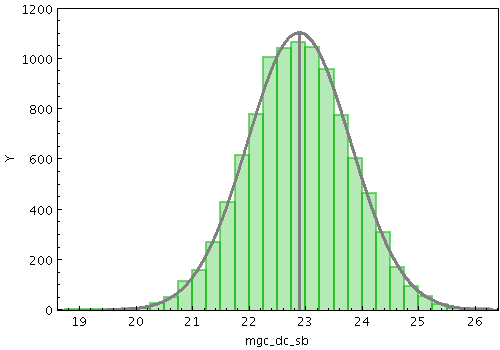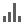Next Previous Up Contents
Up: Plot Forms
Previous: Densogram Form

#### A.4.5.25 Gaussian Form

The Gaussian form () plots a best fit Gaussian to the histogram of a sample of data. In fact, all it does is to calculate the mean and standard deviation of the sample, and plot the corresponding Gaussian curve. The mean and standard deviation values are reported by the plot (see below).Example Gaussian plotGaussian fit configuration panel

These options always appear in the form configuration panel:

Show Mean
If true, this will cause the mean value to be indicated by a vertical line.
Thickness
Controls line thickness.
Dash
Controls the dash pattern of the line (solid, dots, dashes etc).
Antialiasing
If true, lines are antialiased, which makes them look smoother on the screen or bitmapped export images. Has no effect on vector export images (PDF, SVG, EPS).
And these options appear in the form configuration panel for the Plane window, or the Bins control () for the Histogram window:
Normalise
Defines how the histogram is scaled vertically to map its height to data coordinates. The normalisation options match those for the histogram form, so that if the same normalisation and bin size is chosen here, the plotted curve will be a best fit to the shape of the corresponding histogram bars.
Bin Size
Defines the notional size of the bins of a histogram which the plotted Gaussian should match. This option is used only to affect the vertical scaling, and only has effect for certain values of the Normalise option.

As well as drawing the line onto the plot, the calculated fitting coefficients are displayed at the bottom of the form configuration panel, under the heading Report. Note the coefficients are calculated by subset, and are only displayed for one subset at a time. To see the calculated values, select the subset of interest in the Subset selector. The reported items are:

Mean
The mean of the data set.
Standard Deviation
The standard deviation of the data set.
Factor
The scaling factor applied to the basic exponential function to yield the actual function plotted in data coordinates.
Function
The actual function plotted; this includes the numeric values shown by the other report items, and defines exactly what they mean. This expression uses topcat's expression language, and can be used (for instance) directly in the Function plotter.

Next Previous Up Contents